# Influencing factors of peridynamics analysis and calculation

Influencing factors of peridynamics analysis and calculation

Songrong Qian Shuijie Qin*Hongshun Shi

College of Mechanical Engineering, Guizhou University, Guizhou, 550000, China

Key Laboratory of Advanced Manufacturing Technology (Guizhou University), Ministry of Education, Guizhou, 550000, China

Corresponding Author Email:
Shuijie_qin@sina.com
Page:
398-402
|
DOI:
https://doi.org/10.18280/ijht.350224
|
Accepted:
|
Published:
30 June 2017
| Citation

OPEN ACCESS

Abstract:

In this paper, a material structural model is constructed based on a cubic lattice, and applied with a fixed load and an impact load. To provide the basis for parameter selection in modelling, the error and efficiency of structural calculation are analyzed by changing the two parameters in the peridynamics (PD) computation model: near-field region radius δ and m value. During modelling, when δ is given a fixed value, the calculation accuracy will increase with the m value, but the calculation efficiency will change in the opposite trend, thus affecting the path and direction of crack propagation; when m is given a fixed value, the number of point units in the near-field region and the calculation accuracy will not change with δ, but will still affect the path and direction of crack propagation.

Keywords:

peridynamics, modelling, near-field region radius δ, analysis and calculation

1. Introduction

The peridynamics (PD) theory was presented in 2000 by American Professor Silling  of Sandia National Laboratories. Since its invention, the theory has evoked great repercussions in the academic circles, attracting many scholars into the research of PD. The past decade has witnessed the development of PD from infancy to maturity. Today, the theory is particularly mature in terms of the strength analysis of brittle materials.

The PD theory has both advantages and disadvantages. On the one hand, PD analysis and calculation can simulate and analyze deformation, damage and fracture of the material and structure without requiring the object to be continuous or uniform, i.e., the object does not have to be composed of the same material; on the other hand, as one of the biggest obstacles to the application of PD theory [1, 2, 3, 5] , the results of PD calculation are greatly affected by the calculation efficiency andaccuracy.

2. Construction of Structural Model

According to the numerical calculation method of PD theory, it is necessary to separate the analytic target into a number of cubes known as point units [2, 6]. A 3D model should abide by the following separation rules:

(1) The point units feature cubic structure, equal volume and simple structure; the cubic structure ensures the isotropy in the three directions (x, y, z) and facilitates simulation calculation.

## 1a.png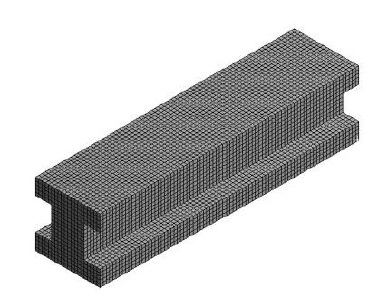(a) 3D discrete model

## 1b.png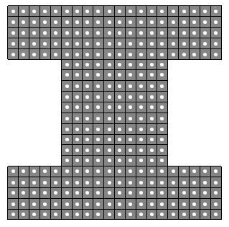(b)Sectional discrete model

Figure 1. Separation of the I-beam structure

(2) The center of the cube is taken as the material point of the calculation, which contains physical information like performance, volume, location, displacement, acceleration, damage, energy, etc.

(3) The two important modelling parameters, δ (near-field region radius) and m value (m=δ/Δx), have a direct impact to the calculation accuracy and efficiency. Hence, the values of these parameters should be determined properly in light of the actual conditions.

In this paper, the I-beam structure is taken as an example. (Figure 1) The parameters required for the constitutive force function are obtained by separating the whole I-beam structure evenly into same sized cubes, and replacing the physical information (performance, volume, location, displacement, acceleration, energy, and damage) of the whole cubic lattice with solid white dots.

3. Setting of Boundary Conditions

There are two types of boundary conditions: loads (force, speed, displacement, etc.) and constraints. In PD theory[7,8,9], the boundary conditions are configured easily by setting the loads and constraints of the point units of a certain area on the structural model[10,11,12].

The boundary conditions, such as those for the cross section in Figure 2, should be configured according to the following principles:

(1) If the boundary area is divided into 2 or more layers, the constrained or loaded area must also have at least 2 layers.

(2) 8 kinds of constraints are provided: x, y, z, xy, yz, xz, xyz and no constraint;

## 2.pngFigure 2. Setting of boundary conditions for the I-beam structure

4. The Flow of Numerical Calculation Program

According to the PD theory, the author firstly configures the boundary conditions, and then solves the displacement, speed, damage and other factors of each point unit by the motion equation [14, 15, 16, 20, 21]. The author also controls the number of the iterative steps (i.e. the number of time steps in iteration) in light of the actual conditions. The calculation results are exported through C language programming. After the location of each point unit is expressed in the form of coordinates, the author exports the contents required for the research selectively, and imports the corresponding coordinates into MATLAB for simulation, thus obtaining the fracture conditions at different time steps. The flow chart of C language programming is shown in Figure 3.

## 3.png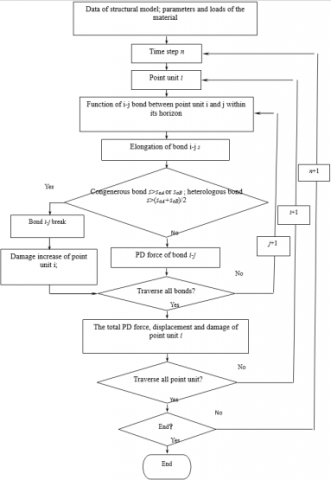Figure 3. Flowchart of C language programming

5. Numerical Calculation Error Analysis

As two importance parameters of PD numerical calculation, δ (near-field region radius) and m value (m=δ/Δx) have a direct influence on the calculation accuracy and efficiency. An equation can be established between them and the traditional Piola-Kirchhoff stress tensor σ[5,17,18,19]:

$\sigma_{n}=\tau(x, n)$      (1)

The surface force density (also known as stress) in PD theory equals the stress in the calculation of traditional mechanical theory. The equation helps compare the effect of δ and m on the efficiency and error of the calculation results.

Analysis of the effect of δ value on calculation error:

Suppose the elastic modulus of the material E = 72 GPa, and the Poisson’s ratio is 0.25,  and let the strain be:

$\varepsilon=\left\{\begin{array}{lll}{e_{11}} & {e_{12}} & {e_{13}} \\ {e_{21}} & {e_{22}} & {e_{23}} \\ {e_{31}} & {e_{32}} & {e_{33}}\end{array}\right\}=\left\{\begin{array}{ccc}{8 \times 10^{-4}} & {0} & {0} \\ {0} & {4.5 \times 10^{-4}} & {0} \\ {0} & {0} & {1.32 \times 10^{-3}}\end{array}\right\}$       (2)

Obtain the theoretical stress value (MPa) by the traditional theory:

$\sigma=E\left\{\begin{array}{ccc}{2 u e_{11}+\lambda\left(e_{11}+e_{22}+e_{33}\right)} & {2 u e_{12}} & {2 u e_{13}} \\ {2 u e_{21}} & {2 u e_{22}+\lambda\left(e_{11}+e_{22}+e_{33}\right)} & {2 u e_{23}} \\ {2 u e_{31}} & {2 u e_{32}} & {2 u e_{33}+\lambda\left(e_{11}+e_{22}+e_{33}\right)}\end{array}\right\}$      (3)

where $u=\frac{E}{(2+2 v)}$ , $\lambda=\frac{E v}{(1-2 v)(1+v)}$ , v is the Poisson’s ratio. Hence:

$\sigma=\left\{\begin{array}{ccc}{120} & {0} & {0} \\ {0} & {100} & {0} \\ {0} & {0} & {150}\end{array}\right\}$       (4)

Therefore, the stress in each direction is respectively σx=120MPa, σy=100MPa and σz=150MPa. Taking the m=δ/Δx =3 as an example, the surface force density τ(x, n) of point x along the direction of n:

$\boldsymbol{\tau}(\mathbf{x}, \mathbf{n})=\int_{L} d \hat{l} \int_{R^{+}} \mathbf{f}\left(\mathbf{u}^{\prime}-\hat{\mathbf{u}}, \mathbf{x}^{\prime}-\hat{\mathbf{x}}\right) d V_{\mathbf{x}^{\prime}}$        (5)

The surface force density is calculated by PD theory for different parameter conditions. The following groups of parameter conditions are selected, and the corresponding stress densities (stress value) are obtained.

(1) Parameter condition 1: m=3, Δx=6mm, δ=18mm

$\sigma=\left\{\begin{array}{ccc}{103.48} & {9.38 \times 10^{-16}} & {-2.25 \times 10^{-15}} \\ {1.17 \times 10^{-16}} & {89.07} & {-3.51 \times 10^{-16}} \\ {9.22 \times 10^{-16}} & {1.86 \times 10^{-15}} & {124.90}\end{array}\right\}$

(2) Parameter condition 2: m=3, Δx=3mm, δ=9mm

$\sigma=\left\{\begin{array}{ccc}{103.48} & {9.38 \times 10^{-16}} & {-2.25 \times 10^{-15}} \\ {1.17 \times 10^{-16}} & {89.07} & {-3.51 \times 10^{-16}} \\ {9.22 \times 10^{-16}} & {1.86 \times 10^{-15}} & {124.90}\end{array}\right\}$

(3) Parameter condition 3: m=3, Δx=1mm, δ=3mm

$\sigma=\left\{\begin{array}{ccc}{102.69} & {-5.56 \times 10^{-16}} & {4.58 \times 10^{-15}} \\ {-3.71 \times 10^{-17}} & {88.38} & {1.84 \times 10^{-15}} \\ {9.68 \times 10^{-16}} & {1.47 \times 10^{-15}} & {123.97}\end{array}\right\}$

(4) Parameter condition 4: m=3, Δx=0.1mm, δ=0.3mm

$\sigma=\left\{\begin{array}{rcc}{102.62} & {2.78 \times 10^{-15}} & {6.74 \times 10^{-15}} \\ {-3.30 \times 10^{-16}} & {88.34} & {1.13 \times 10^{-15}} \\ {3.11 \times 10^{-15}} & {-1.02 \times 10^{-15}} & {123.87}\end{array}\right\}$

(5) Parameter condition 5: m=3, Δx=0.05mm, δ=0.15mm

$\sigma=\left\{\begin{array}{ccc}{102.62} & {2.78 \times 10^{-15}} & {6.74 \times 10^{-15}} \\ {-3.30 \times 10^{-16}} & {88.34} & {1.13 \times 10^{-15}} \\ {3.11 \times 10^{-15}} & {-1.02 \times 10^{-15}} & {123.87}\end{array}\right\}$

The comparison between the results of surface force density in PD theory and the results obtained by traditional mechanical theory (Figure 4) show that: when m is given a fixed value, the PD theory results are almost invariant to the changes in the side length of point unit and near-field region, but there exists some differences between the results and the results of the traditional theory, the calculation efficiency, however, remains the same because the number of point units is unchanged.

## 4a.png(a)σx

## 4b.png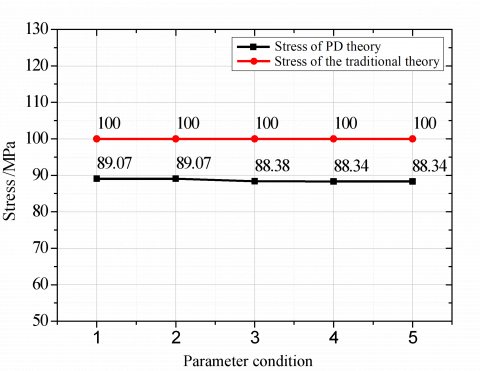(b) σy

## 4c.png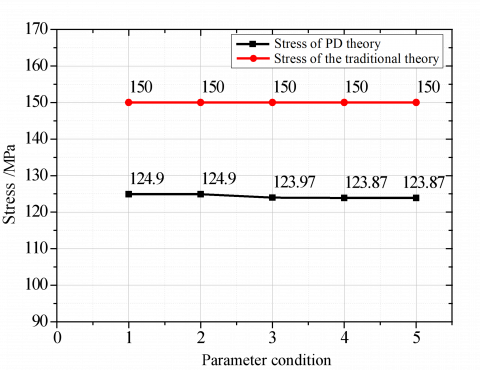(c) σz

Figure 4. Comparison between the stress in each of the three directions obtained by PD theory and those obtained by the traditional theory at different parameter conditions (m=3)

In similar manner, the effect of m value on calculation error is analyzed. Suppose the elastic modulus of the material E = 72 GPa, and the Poisson’s ratio is 0.25, and let the strain be:

$\varepsilon=\left\{\begin{array}{ccc}{8 \times 10^{-4}} & {0} & {0} \\ {0} & {4.5 \times 10^{-4}} & {0} \\ {0} & {0} & {1.32 \times 10^{-3}}\end{array}\right\}$

Obtain the theoretical stress value (MPa) by the traditional theory:

$\sigma=\left\{\begin{array}{ccc}{120} & {0} & {0} \\ {0} & {100} & {0} \\ {0} & {0} & {150}\end{array}\right\}$

Therefore, the stress in each direction is respectively σx=120MPa, σy=100MPa and σz=150MPa. Let δ be 3mm, and calculate the surface force density by PD theory at different m values. The following groups of m values are selected, and the corresponding stress densities (stress value) are obtained.

(1) Parameter condition 1: m=2, Δx=1.5mm, δ=3mm

$\sigma=\left\{\begin{array}{ccc}{100.41} & {1.17 \times 10^{-15}} & {9.90 \times 10^{-15}} \\ {2.84 \times 10^{-15}} & {85.08} & {1.54 \times 10^{-14}} \\ {3.90 \times 10^{-15}} & {-3.07 \times 10^{-15}} & {123.19}\end{array}\right\}$

(2) Parameter condition 2: m=3, Δx=1mm, δ=3mm

$\sigma=\left\{\begin{array}{ccc}{102.69} & {-5.56 \times 10^{-16}} & {4.58 \times 10^{-15}} \\ {-3.71 \times 10^{-17}} & {88.38} & {1.84 \times 10^{-15}} \\ {9.68 \times 10^{-16}} & {1.47 \times 10^{-15}} & {123.97}\end{array}\right]$

(3) Parameter condition 3: m=4, Δx=0.75mm, δ=3mm

$\sigma=\left\{\begin{array}{ccc}{105.64} & {-1.91 \times 10^{-18}} & {1.31 \times 10^{-15}} \\ {1.67 \times 10^{-16}} & {90.74} & {4.18 \times 10^{-15}} \\ {-7.56 \times 10^{-16}} & {-2.34 \times 10^{-15}} & {127.80}\end{array}\right\}$

(4) Parameter condition 4: m=6, Δx=0.5mm, δ=3mm

$\sigma=\left\{\begin{array}{ccc}{109.43} & {-4.17 \times 10^{-16}} & {-1.84 \times 10^{-15}} \\ {-9.61 \times 10^{-17}} & {93.05} & {2.97 \times 10^{-16}} \\ {3.82 \times 10^{-17}} & {1.81 \times 10^{-15}} & {133.79}\end{array}\right\}$

(5) Parameter condition 5: m=10, Δx=0.3mm, δ=3mm

$\sigma=\left\{\begin{array}{ccc}{110.06} & {-7.72 \times 10^{-17}} & {8.47 \times 10^{-16}} \\ {8.32 \times 10^{-17}} & {92.85} & {6.55 \times 10^{-16}} \\ {-1.26 \times 10^{-16}} & {1.65 \times 10^{-16}} & {135.64}\end{array}\right]$

(6) Parameter condition 6: m=15, Δx=0.2mm, δ=3mm

$\sigma=\left\{\begin{array}{rrr}{114.68} & {-5.57 \times 10^{-17}} & {-1.58 \times 10^{-16}} \\ {-1.28 \times 10^{-17}} & {96.37} & {-1.21 \times 10^{-16}} \\ {-6.53 \times 10^{-18}} & {-4.99 \times 10^{-17}} & {141.90}\end{array}\right\}$

(7) Parameter condition 7: m=60, Δx=0.05mm, δ=3mm

$\sigma=\left\{\begin{array}{ccc}{115.42} & {8.28 \times 10^{-19}} & {2.61 \times 10^{-17}} \\ {9.46 \times 10^{-19}} & {96.07} & {5.27 \times 10^{-18}} \\ {2.89 \times 10^{-18}} & {-2.52 \times 10^{-17}} & {144.21}\end{array}\right\}$

It is demonstrated by the comparison between the results of surface force density in PD theory and the results obtained by traditional mechanical theory (Figure 5) that: when δ is given a fixed value, the stress will increase and move closer to the results of the traditional theory as the m value increases, i.e. the number of point units in the near-field region rises. Thus, the calculation accuracy grows with the m value; In contrast, the calculation efficiency declines because the number of point units in the near-field region of each point unit increases while the total number of point units remain the same.

## 5a.png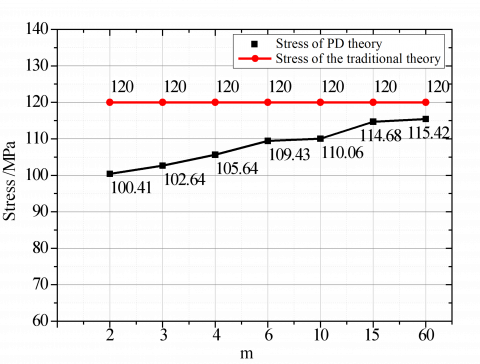(a)σx

## 5b.png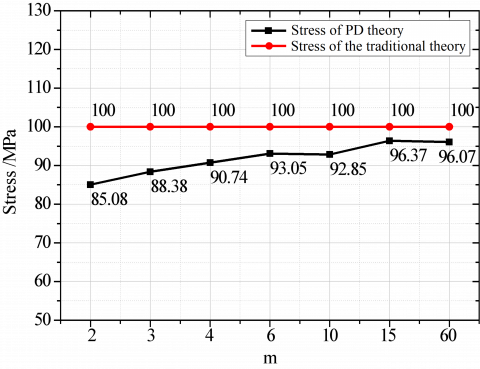(b) σy

## 5c.png(c) σz

Figure 5. Comparison between the stress in each of the three directions obtained by PD theory and those obtained by the traditional theory at different m values (δ=3mm)

Through the above analysis, the result of m=δ/△x influence on calculation accuracy, the ‘m’value, the greater  accuracy is higher, but the computation efficiency is reduced, in order to ensure the efficiency and precision of ‘m’ value between 3-5 is good.

6. Conclusions

This paper introduces the modeling method for the object, that is, to construct an analysis model based on a unified cubic lattice, analyzes how to set up the initial PD conditions under fixed load and impact load, explains C language numerical calculation and simulation, and draws the flowchart of simulation calculation. The optimal range of modeling parameters are offered through analysis of the influence of near-field region radius δ and m value over error and efficiency, especially their impacts to the results of numerical simulation. It is discovered that, when δ is given a fixed value during the modelling process, the calculation accuracy will increase with the m value, but the calculation efficiency will

change in the opposite trend, thus affecting the path and direction of crack propagation; when m is given a fixed value and δ is allowed to change, the number of point units in the near-field region and the calculation accuracy will not change, but will still affect the path and direction of crack propagation. As a result, special attention should be paid to materials containing defects (cracks, pores) by taking reasonable m values and δ values in light of the actual conditions of the material (defect size, material performance, and material composition); For materials with no defect, neither m value nor δ value has significant impact to the path and direction of crack propagation. In this case, small m value and large δ value should be adopted to improve calculation efficiency.

Acknowledgment

Funded by the Joint Funds of Guizhou Province (Guizhou/Science/Cooperation LH  No. 7624); Guizhou Provincial Foundation for Scientific Activities of Returned Scholars (Guizhou/Human/Capital  No. 13).

References

 Silling S.A. (2000). Reformulation of elasticity theory for discontinuities and long-range forces, Journal of the Mechanics and Physics of Solids, Vol. 48, No. 1, pp. 175-209. DOI: 10.1016/S0022-5096(99)00029-0

 Silling S.A. (2002). Peridynamic modeling of the failure of heterogeneous solids, Report on ARO Workshop on Analysis and Design of New Engineered Materials and Systems with Applications, Albuquerque, US.

 Belytschko T., Lu Y.Y., Gu L. (1995). Crack propagation by element-free Galerkin methods, Engineering Fracture Mechanics, Vol. 51, No. 2, pp. 295-315. DOI: 10.1016/0013-7944(94)00153-9

 Belytschko T., Black T. (1999). Elastic crack growth in finite elements with minimal remeshing, International Journal for Numerical Method in Engineering, Vol. 45, No. 5, pp. 601-620.

 Bobaru F., Hu W.K. (2012). The meaning, selection, and use of the peridynamic horizon and its relation to crack branching in brittle materials, International Journal of Fracture, Vol. 176, No. 2, pp. 215–222. DOI: 10.1007/s10704-012-9725-z

 Bobaru F., Zhang G.F. (2015). Why do cracks branch: a peridynamic investigation of dynamic brittle fracture, International Journal of Fracture, Vol. 196, No. 1-2, pp. 59-98. DOI: 10.1007/s10704-015-0056-8

 Brock D. (2008). Elementary Engineering Fracture Mechanics, 4th edition, Springer, Berlin.

 Gerstle W., Sau N., Silling S. (2007). Peridynamic modeling of concrete structures, Nuclear Engineering and Design, Vol. 237, No. 12-13, pp. 1250-1258. DOI: 10.1016/j.nucengdes.2006.10.002

 Ha Y.D., Bobaru F. (2010). Studies of dynamic crack propagation and crack branching with peridynamics, International Journal of Fracture, Vol. 162, No. 1, pp. 229–244. DOI: 10.1007/s10704-010-9442-4

 Huang D., Zhang Q., Qiao P., Shen F. (2010). A review on peridynamics (PD) method and its applications, Advances in Mechanics, Vol. 40, No. 4, pp. 448-459.

 Kikic B., Madenc E. (2009). Structural stability and failure analysis using peridynamic theory, International Journal of Non-linear Mechanics, Vol. 44, No. 8, pp. 845-854. DOI: 10.1016/j.ijnonlinmec.2009.05.007

 Kilic B. (2008). Peridynamic theory for progressive failure prediction in homogeneous and heterogeneous materials, Dissertations & Theses, Gradworks.

 Silling S.A., Weckner O., Askari E., Bobaru F. (2010). Crack nucleation in a peridynamic solid, International Journal of Fracture, Vol. 162, No. 1, pp. 219–227. DOI: 10.1007/s10704-010-9447-z

 Silling S.A., Zimmermann M., Abeyaratine R. (2003). Deformation of a peridynamic bar, Journal of Elasticity, Vol. 73, No. 1, pp. 173-190. DOI: 10.1023/B: ELAS.0000029931.03844.4f

 Yu K.B. (2011). Enhanced integration method for the peridynamic theory, Shanghai Jiao Tong University, Shanghai.

 Bounaouara H., Ettouati H., Ticha H.B., Mhimid A., Sautet J.C. (2015). Numerical simulation of gas-particles two phase flow in pipe of complex geometry: pneumatic conveying of olive cake particles toward a dust burner, International Journal of Heat and Technology, Vol. 33, No. 1, pp. 91-98. DOI: 10.18280/ijht.330114

 Mahmoudi A., Mejri I. (2015). Isothermal carbonization of wood particle: application of the Lattice Boltzmann Method, International Journal of Heat and Technology, Vol. 33, No. 2, pp. 129-134. DOI: 10.18280/ijht.330221

 Mansouri Z., Aouissi M., Boushaki T. (2016). A numerical study of swirl effects on the flow and flame dynamics in a lean premixed combustor, International Journal of Heat and Technology, Vol. 34, No. 2, pp. 227-235. DOI: 10.18280/ijht.340211

 Aissa A., Said B., Mohamed T., Ahmed B. (2015). Numerical study of mixed convection in cylindrical Czochralski configuration for crystal growth of silicon, International Journal of Heat and Technology, Vol. 33, No. 1, pp. 39-46. DOI: 10.18280/ijht.330106

 Ansari M.M., Chakrabarti A., Iqbal M.A. (2016). Effects of impactor and other geometric parameters on impact behavior of FRP laminated composite plate, Modelling, Measurement and Control A, Vol. 89, No. 1, pp. 25-44

 Guo Z., Zhang J.S., Zheng C.M., Sun Z.C. (2016). Dynamic performance analysis of the induction motor drive fed by current-source based on Ansoft, Modelling, Measurement and Control A, Vol. 89, No. 1, pp. 118-129.